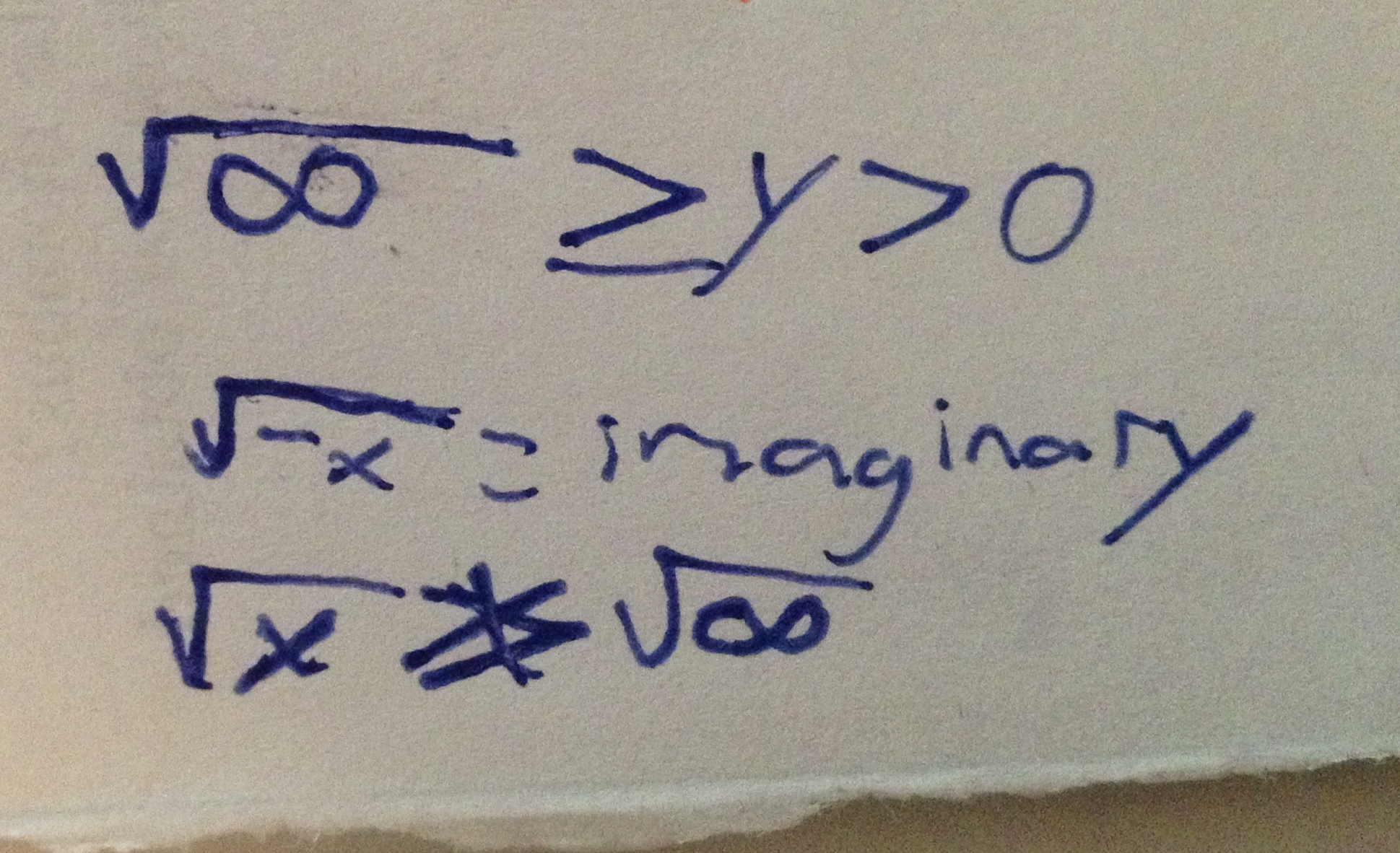Categories

# Infinity!The question asked for the range of the function SQRT(x).  What are your thoughts about the way the student answered the question. What does it show about what this particular student knows.*

In the past, I’ve publicly kvetched about how the only questions that we grapple with on this blog are about the particularities, rather than the generalities, of student work. This is a time when I think that the most interesting questions are accessed through thinking about what this particular kid was thinking. I’d also be interested in hearing how you think this represents a trend in students’ thinking, in general.

Thanks to Tina for the submission!

## 5 replies on “Infinity!”

Hmmm, my first reaction is to wish that the closed interval on the compound inequality was with the zero rather than with the square root of infinity. I am pretty certain that this is purely mechanical work, not conceptual in their thinking about infinity in any way. The simple question of range is (fairly well) attacked in that first inequality and then things get odd form there. The negative x is an unfortunate, but common, mistake where a negative sign automatically means a negative number. So that would explain the sqrt of negative x being an automatic imaginary number. The last line? Lost there.
As a follow up I would touch on the fact that negative x is not necessarily a negative number. Discuss the idea that the range is a question only appropriate on the domain so the negative x business is not really part of the conversation anyway.

Good point about the -x. I used the shorthand sqrt(-) and sqrt(+) in discussion. It would be interesting to have students sort out the difference in notation.

Michael Paul Goldenbergsays:

Interesting. Was this something students had been taught previously and were now being assessed upon (even informally)? Or was it a general idea they’d learned about domains of functions of real variables and were now being asked to apply that to a new case, namely sqr(x)?

Substituting infinity for x is problematic because it’s not a real number or even a complex number. But the student may have seen other domains in which positive (and/or) negative infinity were upper/lower bounds used in inequalities to express the domain. So perhaps the thought was to simply stick in pos infinity like it was a number to see what came out. The conclusion, interestingly, is that sqr(infinity) is still greater than any non-zero positive number y. And that’s true.

The student also knows that the square root of any non-negative number is positive, hence greater than zero. So s/he is bounding SOMETHING (a bit unclear whether it is the input or output because of the use of ‘y’)between 0 and infinity, but of course the direction of the inequality is non-standard and the inequality symbols should be swapped, so that 0 is included and infinity isn’t.

The last line is amusing but not clearly written enough to be very sure what the intent is.

I think there are sensible elements in what is written, mixed in with nonsense, so that more problems or a conversation with the student would be necessary to separate out what the student believes in fact (rightly or wrongly) vs. what is just an artifact of scribbled notation.

As for what general trend in student thinking might be represented, nothing immediately comes to mind. There’s not much new about kids or adults writing nonsense mathematics due to confusion about both notation and meaning, as far as I am aware. But I would love to ask questions of this student.

This is an early quiz in PreCalc after they had a summer assignment on graph, domain and range of 8 parent functions. I didn’t mention anything about calculating range by substituting x values but it’s intriguing that’s the approach this student took.

The usual style for writing functions at school is to assume the domain of a real function is all the real numbers unless stated otherwise. But this leads to a tendency to forget to state the domain explicitly every time, even when it needs to be stated, and that leads to lack of awareness of domain restrictions that are important.

The student’s problems are made worse by teaching that introduces a symbol for infinity and gives it the appearance of a number combined with some weird properties that a number would not have.

I probably would have offered this as an answer:

dom[SQRT] = { x : R | x >= 0 }

having learned to define SQRT with something like:

forall x : R | x >= 0 * (SQRT[x])^2 = x

Errors like this are primarily a consequence of the design of the mathematics and its notation, and only secondly down to teaching and student stupidity.